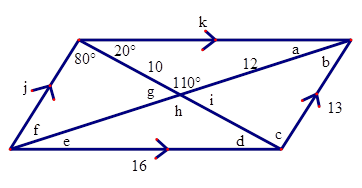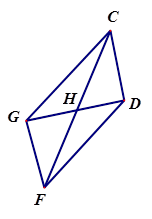# Parallelograms Warm Up

Author:
powere

## Use the diagram below to fill in for the missing lengths and angles. Please ignore the units when entering an answer.1. A = _____ ﻿B = _____ ﻿C = _____ ﻿D = _____ ﻿ E = _____ ﻿ F = _____ ﻿G = _____ ﻿H = _____ ﻿ I = _____ ﻿ J = _____ ﻿K = _____2. CH = ____3. GF is parallel to _____4. <DCG is congruent to _____5. DC = _____6. <DCG is supplementary to ____ and ____7. Triangle HGC is congruent to _____